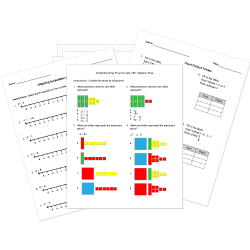Looking for Algebra worksheets?
Check out our pre-made Algebra worksheets!
 Tweet##### Browse Questions
• Arts (658)
• English Language Arts (3081)
• English as a Second Language ESL (1429)
• Health and Medicine (721)
• Life Skills (476)
• Math (2471)

• ### Systems of Equations

• #### Trigonometry

• Physical Education (420)
• Science (7122)
• Social Studies (2157)
• Study Skills and Strategies (13)
• Technology (485)
• Vocational Education (1000)

You can create printable tests and worksheets from these Grade 10 Function and Algebra Concepts questions! Select one or more questions using the checkboxes above each question. Then click the add selected questions to a test button before moving to another page.

Previous Next
Grade 10 Systems of Equations CCSS: HSA-CED.A.3, HSA-REI.C.6
If you pay $11 for 1 apple and 9 bananas and pay$7 for 3 apples and 1 banana, how much does an apple and a banana cost?
1. Apple = $-1 Banana =$2/3
2. Apple = $2 Banana =$1
3. Apple = $1 Banana =$4/9
4. Apple = $1/2 Banana =$1/2
Factor. $6x^2 - 19x + 10$
1. $(3x-10)(2x+1)$
2. $(6x - 5)(x-2)$
3. $(3x - 5)(2x-2)$
4. $(3x-2)(2x-5)$
Grade 10 Systems of Equations CCSS: HSA-REI.C.6
Grade 10 Polynomials and Rational Expressions
Match each factored expression with its corresponding expanded form.
 __ $2(x-5)^2$ A. $3x^3-27x^2$ __ $3x^2(x-9)$ B. $2x^2 + 2x-24$ __ $2(x+4)(x-3)$ C. $x^3-x^2-5x+5$ __ $(x^2-5)(x-1)$ D. $2x^2 -20x+50$
Grade 10 Functions and Relations CCSS: HSF-IF.A.1
Is the following a function? Explain why or why not.1. Yes, this does represent a function, because all the points are distinct (none of them are coincident).
2. No, this does not represent a function, since a function can not be made up of only points.
3. No, this does not represent a function, since it fails the vertical line test.
4. No, this does not represent a function, since a function is made up of many more points.
Grade 10 Literal Equations CCSS: HSA-CED.A.4
Solve for $B$.

$A=BC$
1. $B=AC$
2. $B=C/A$
3. $B=A/C$
4. $A=B/C$
Grade 10 Literal Equations CCSS: HSA-CED.A.4
Solve for $z$.

$x=z/y$
1. $z=xy$
2. $z=x/y$
3. $z=y/x$
4. $x=zy$
Grade 10 Literal Equations CCSS: HSA-CED.A.4
Solve for $r$.

$4x+y=3t-2r$
1. $(4x+y-3t)/-2=r$
2. $(4x+y-3t)/2=r$
3. $(4x+y)/6t=r$
4. $-2(4x+y)/3t=r$
Grade 10 Literal Equations CCSS: HSA-CED.A.4
Solve for $r$.

$a=v^2/r$
1. $r=a/v^2$
2. $r=v^2a$
3. $r=v^2/a$
4. $r=sqrt(v)/a$
Grade 10 Systems of Equations CCSS: HSA-REI.C.6
Solve the system of equations.
$y=x-8$
$y=-3x$
1. $(2,-6)$
2. $(-2,6)$
3. $(3,-9)$
4. $(1,7)$
Grade 10 Systems of Equations CCSS: HSA-REI.C.6
Select the solution to the system of linear equations.
$x+y=8$
$2x-y=1$
1. $(5, 2)$
2. $(2/5, 2/11)$
3. $(2, 5)$
4. $(3, 5)$
Write $64 ^(1/2) = 8$ in logarithmic form.
1. $log_64 (1/2) =8$
2. $log_(1/2) 8 =64$
3. $log_64 8 = 1/2$
4. $log_(1/2) 64 =8$
By factoring the expression $12x^2 + 6x - 6$, find the zeros of the quadratic function this expression defines.
1. $x=-1, 1/2$
2. $x = -3/4, 2/3$
3. $x = -3/2, 1/3$
4. $x = -2, 1/4$
Grade 10 Functions and Relations CCSS: HSF-IF.A.2
Evaluate $f(x) = -x + 5$ for $x = -2$.
1. $f(-2) = 7$
2. $f(-2) = 10$
3. $f(-2) = -7$
4. $f(-2) = 3$
Grade 10 Systems of Equations CCSS: HSA-REI.C.6
Grade 10 Systems of Equations CCSS: HSA-REI.C.6
Select the solution to the system of linear equations.
$x+2y=11$
$3x-2y=9$
1. $(3, 2)$
2. $(5, 3)$
3. $(4.5, 2)$
4. $(1/2, 2/3)$
Grade 10 Functions and Relations CCSS: HSF-IF.A.2
Evaluate $f(x) = -5x$ for $x = 0$.
1. $f(0) = -5$
2. $f(0) = 0$
3. $f(0) = 5$
4. $f(0) = 1$
Previous Next
You need to have at least 5 reputation to vote a question down. Learn How To Earn Badges.